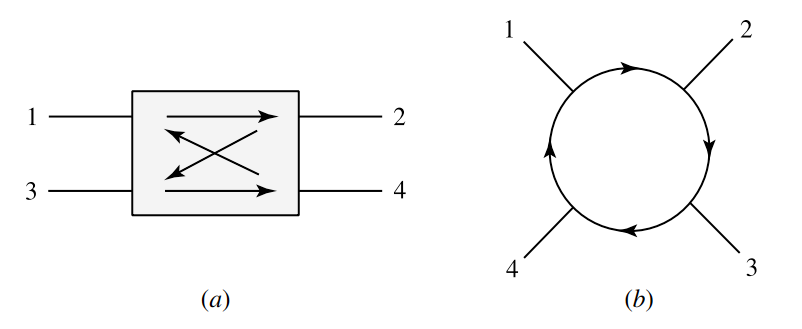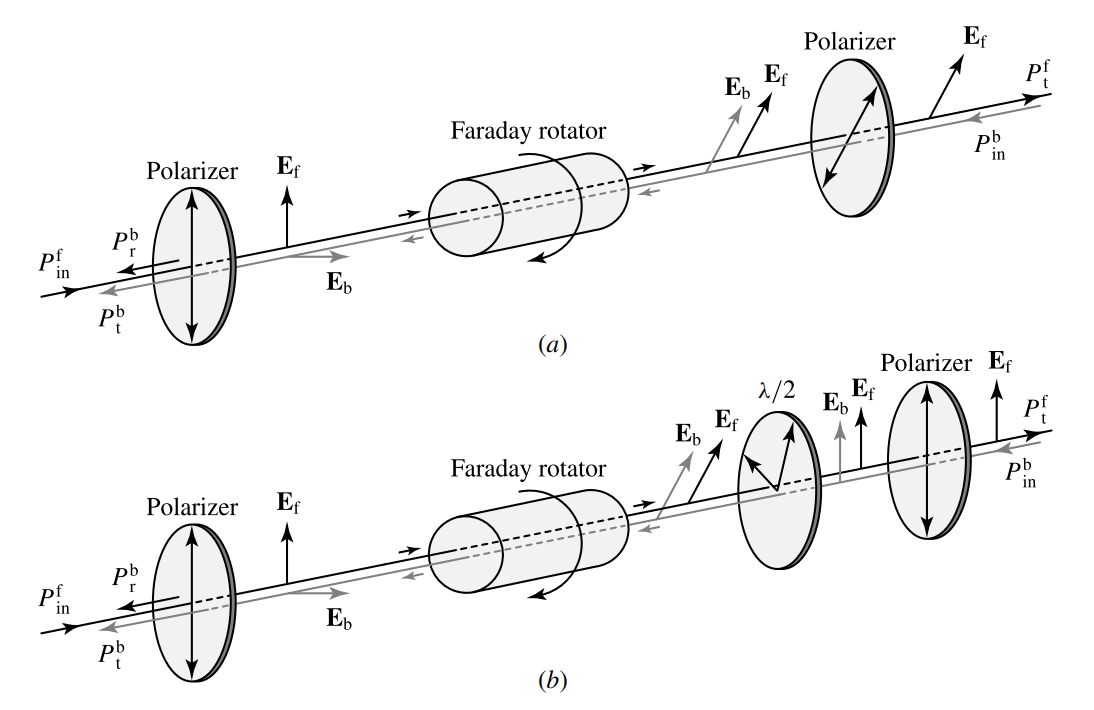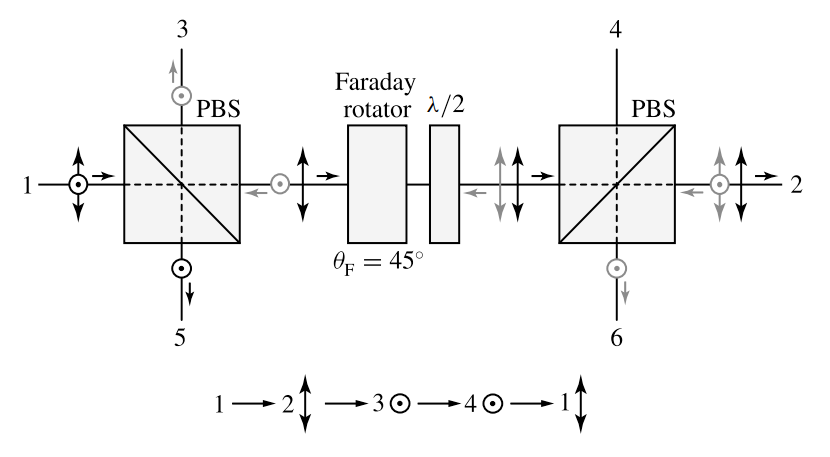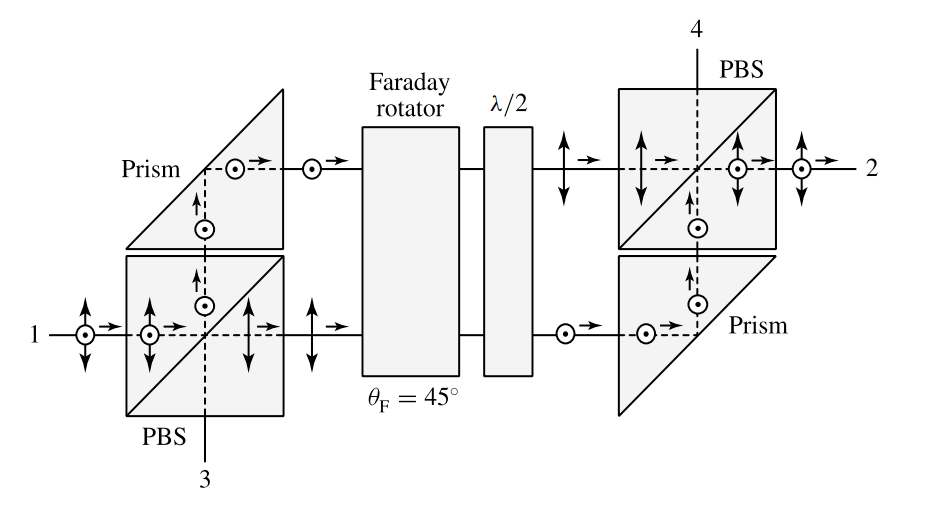# Optical Isolators and Circulators

This is continuation from the previous tutorial - magneto-optic Kerr effect.

In an optical system, reflections and backscattering of light often cause serious problems ranging from noise in the photodetectors to instabilities in the light sources. A feedback, even at an extremely low level, to a laser usually has a significant effect on the laser characteristics. It can change the laser frequency, increase the laser noise, create fluctuations in the laser intensity, lock the laser to a different mode of operation, or drive the laser into instability, even chaos. A feedback to a photodetector or other parts of an optical system also has many undesirable effects. Sometimes the problem is so severe that it renders the entire system useless.

Optical isolators are needed to avoid such problems. An optical isolator is a nonreciprocal device that transmits an optical wave in one direction but blocks it in the reverse direction much as the function of a diode in an electric circuit. The key specifications of an optical isolator are the insertion loss, the return loss, and the reverse isolation of the device.Figure 7-4. Diagrammatic illustration of an optical isolator with (a) a forward-propagating input signal, $$P_\text{in}^\text{f}$$, and (b) a backward-propagating input signal, $$P_\text{in}^\text{b}$$, together with the transmitted and reflected components for defining the key specifications.

The insertion loss is the attenuation of an optical signal propagating in the forward direction through the optical isolator. The return loss specifies how well the reflection of the forward-propagating signal is eliminated. Referring to figure 7-4(a), they are defined as

$\tag{7-39}\text{Insetion loss}=-10\log\frac{P_\text{t}^\text{f}}{P_\text{in}^\text{f}}$

$\tag{7-40}\text{Return loss}=-10\log\frac{P_\text{r}^\text{b}}{P_\text{in}^\text{f}}$

The reverse isolation is a measure of the isolation function of the device and is defined as the attenuation of a backward-propagating optical signal through the isolator. Referring to figure 7-4(b), it is given by

$\tag{7-41}\text{Return loss}=-10\log\frac{P_\text{t}^\text{b}}{P_\text{in}^\text{b}}$

For a good isolator, it is desired that the value of the insertion loss be as low as possible while those of the return loss and the reverse isolation be as high as possible. Furthermore, the return loss has to be higher than the reverse isolation for a device to be functionally useful.

In some systems, such as fiber-optic transmission systems, bidirectional transmission with isolation from backscattering and reflections is necessary. This function can be accomplished by an optical circulator, which loops an optical signal through successive ports while blocking backscattered and reflected light.

The diagrams in figure 7-5 illustrate the function of a four-port optical circulator. A true optical circulator connects all ports in an endless loop, as shown in figure 7-5(b). A quasi-optical circulator loops an optical signal through successive ports but is not able to transmit it from the last port to the first port.Figure 7-5. Diagrammatic illustration of (a) a four-port optical circulator and (b) its looping function.

As an example of the application of optical circulators, figure 7-6 shows bidirectional transmission in a single-fiber transmission line using one circulator on each end. Both true circulators and quasi-circulators are acceptable for this particular application.

The key components of optical isolators and circulators are the Faraday rotators because only the Faraday effect has the nonreciprocity in transmission required by these devices.

The mostly commonly used materials for Faraday rotators in these applications are the YIG crystal and bismuth-substituted rare-earth iron garnet films, such as $$\text{Bi}_x\text{Y}_{3-x}\text{Fe}_5\text{O}_{12}(\text{Bi:YIG})$$, $$\text{Gd}_{3-x}\text{Bi}_x\text{Fe}_5\text{O}_{12}$$, $$\text{(YbTbBi)}_3\text{Fe}_5\text{O}_{12}$$, and many other different compositions.

These ferrimagnetic films have very large values of specific Faraday rotation and are used for making very compact isolators and circulators.

Paramagnetic garnets, such as $$\text{Tb}_3\text{Ga}_5\text{O}_{12}\text{(TGG)}$$ and $$\text{Tb}_3\text{Al}_5\text{O}_{12}$$, and paramagnetic glasses, such as terbium-doped glasses, are sometimes also used.

Whether the material used is ferrimagnetic or paramagnetic, it is normally placed in the magnetic field of a permanent magnet. In the case of a ferrimagnetic garnet, the magnetic field keeps the garnet magnetized, preferably at saturation magnetization for maximum efficiency.

Polarization-dependent isolators

The basic structure of an optical isolator consists of a Faraday rotator of total Faraday rotation angle $$\theta_\text{F}=45^\circ$$ and two linear polarizers, as shown in figure 7-7(a).Figure 7-7. Basic structure and principle of polarization-dependent optical isolators. (a) The basic structure of an optical isolator changes the polarization at the output. (b) The addition of a properly oriented half-wave plate in the isolator restores the polarization back to its original direction. $$\lambda/2$$ labels a half-wave plate.

The axis of the input polarizer can be arbitrarily oriented, but the axis of the output polarizer has to be rotated by $$\theta_\text{F}=45^\circ$$ with respect to that of the input one in the same direction as the polarization rotation caused by the Faraday rotator.

An optical wave entering the device in the forward direction through the input polarizer becomes linearly polarized by this polarizer. The Faraday rotator then rotates its plane of polarization by 45° into a direction parallel to the axis of the output polarizer. Therefore, the linearly polarized wave emerging from the Faraday rotator is transmitted by the output polarizer without attenuation.

For reverse isolation, an optical wave of any polarization entering from the output end is polarized by the output polarizer. Because Faraday rotation is independent of wave propagation direction, the backward-propagating wave emerging from the Faraday rotator has a linear polarization orthogonal to the axis of the input polarizer and is thus blocked.

To minimize the insertion loss in the application of this isolator, the input optical wave clearly has to be linearly polarized in a direction parallel to the axis of the input polarizer.

After passing through the isolator, its plane of polarization is rotated by 45° though it is still linearly polarized. Therefore, the basic isolator shown in figure 7-7(a) changes the polarization direction of the optical wave.

This problem can be eliminated by adding a properly oriented half-wave plate and reorienting the output polarizer, as shown in figure 7-7(b). Because the half-wave plate is a reciprocal element, it does not interfere with the function of the isolator while restoring the polarization direction.

For both devices shown in figures 7-7(a) and (b), the function of the reverse isolation is independent of the polarization of the backward-propagating wave.

To obtain a high return loss, the surfaces of every component along the optical path in an isolator have to be antireflection coated. To eliminate any further residual reflection, the facets of the components are sometimes cut or tilted at a small angle, typically somewhere between 1° and 10°.

The antireflection coatings also serve the purpose of reducing the insertion loss. The limiting factor for insertion loss is the absorption of the optical components, particularly that of the Faraday rotator.

That part of the absorption that contributes to circular dichroism also reduces the reverse isolation by making a linearly polarized input wave elliptically polarized after its passage through the rotator.

Therefore, a Faraday rotator that has both a large specific rotation and a low absorption coefficient is most desirable.

In order to have a low insertion loss and a high reverse isolation, it is also very important to choose polarizers of very high extinction ratios. The extinction ratio of a polarizer is an intrinsic property of the polarizer that is defined as

$\tag{7-42}\text{ER}=-10\log\frac{T_\perp}{T_\parallel}=-10\log\sigma$

where $$T_\parallel$$ is the transmittance of an optical wave that is linearly polarized in a direction parallel to the axis of the polarizer, $$T_\perp$$ is that of its orthogonal polarization, and $$\sigma=T_\perp/T_\parallel$$ is the extinction ratio in the linear scale.

Other major factors that affect both the insertion loss and the reverse isolation are the Faraday rotation angle $$\theta_\text{F}$$ and the Faraday ellipticity $$\epsilon_\text{F}$$ generated by the Faraday rotator, as well as the angle $$theta_\text{p}$$ between the axis of the output polarizer and that of the input polarizer.

For an isolator of the basic structure shown in figure 7-7(a), where the input and output polarizers have extinction ratio $$\sigma_\text{in}$$ and $$\sigma_\text{out}$$, respectively, the insertion loss and the reverse isolation can be expressed, respectively, as

$\tag{7-43}\text{Inserton loss}=L_0-10\log[\cos^2(\theta_\text{F}-\theta_\text{p})+\sigma_\text{out}\sin^2(\theta_\text{F}-\theta_\text{p})+(1+\sigma_\text{out})\epsilon_\text{F}^2]$

$\tag{7-44}\text{Reverse isolation}=L_0-10\log[\cos^2(\theta_\text{F}+\theta_\text{p})+\sigma_\text{in}\sin^2(\theta_\text{F}+\theta_\text{p})+(1+\sigma_\text{in})\epsilon_\text{F}^2]$

where $$L_0$$ is the background optical loss including absorption losses of the Faraday rotator and the polarizers and residual reflection losses due to imperfect antireflection coating of the optical surfaces in the system.

It can be seen from (7-43) and (744) that the insertion loss is minimized while the reverse isolation is maximized by choosing $$\theta_\text{F}=\theta_\text{p}=45^\circ$$. The effect on the reverse isolation caused by a deviation of $$\theta_\text{F}$$ from $$45^\circ$$ can be removed by adjusting $$\theta_\text{p}$$ for a counter deviation of the same amount, albeit at the expense of increasing the insertion loss.

Similar relations, with proper modifications according to a particular structure, can be written for the isolation shown in figure 7-7(b), as well as for those of other structures.

Example 7-3

An optical isolator of the structure shown in figure 7-7(a) has a background optical loss of 0.5 dB. Both the input and output polarizers have the same extinction ratio of 40 dB. The Faraday ellipticity generated by the Faraday rotator is negligibly small. If the Faraday rotation angle has the ideal value of $$\theta_\text{F}=45^\circ$$, what are the minimum insertion loss and the maximum reverse isolation? If the Faraday rotation angle is off by only $$1^\circ$$ from this ideal value, what is the reverse isolation when the insertion loss is minimized? What is the insertion loss when the reverse isolation is maximized? Is it better to minimize the insertion loss or to maximize the reverse isolation in this situation?

From (7-42), we find that $$\sigma=10^{-4}$$ for both input and output polarizers of a 40-dB extinction ratio. When $$\theta_\text{F}=45^\circ$$, the minimum insertion loss and the maximum reverse isolation are simultaneously achieved by setting $$\theta_\text{p}=45^\circ$$. Because $$L_0$$ = 0.5 dB and $$\epsilon_\text{F}\approx$$ 0, we find from (7-43) and (7-44) that

Minimum insertion loss = $$L_0$$ = 0.5 dB,

Maximum reverse isolation = $$L_0-10\log\sigma$$ = 40.5 dB.

When the Faraday rotation angle is off by $$1^\circ$$, we have $$\theta_\text{F}=45\pm1^\circ$$. To minimize the insertion loss, we choose $$\theta_\text{p}=\theta_\text{F}=45\pm1^\circ$$ so that $$\theta_\text{F}-\theta_\text{p}=0$$, but then $$\theta_\text{F}+\theta_\text{p}=90\pm2^\circ$$. With this choice of $$\theta_\text{p}$$, the insertion loss remains at its minimum value of 0.5 dB according to (7-43), but the reverse isolation becomes

$L_0-10\log[\cos^2(90^\circ\pm2^\circ)+\sigma\sin^2(90^\circ\pm2^\circ)]\approx29.3\text{ dB}$

To maximize the reverse isolation, we have to choose $$\theta_\text{p}=45\mp1^\circ$$ so that $$\theta_\text{F}+\theta_\text{p}=90^\circ$$, but then $$\theta_\text{F}-\theta_\text{p}=\pm2^\circ$$. With this choice of $$\theta_\text{p}$$, the reverse isolation remains at its maximum value of 40.5 dB according to (7-44), but the insertion loss becomes

$L_0-10\log[\cos^2(\pm2^\circ)+\sigma\sin^2(\pm2^\circ)]=0.505\text{ dB}$

We find that there is a significant reduction of 11.2 dB in the reverse isolation when the insertion loss is minimized, but there is only a negligibly small increase of 0.005 dB in the insertion loss when the reverse isolation is maximized. One clearly should choose to maximize the reverse isolation by maintaining $$\theta_\text{F}+\theta_\text{p}=90^\circ$$ when $$\theta_\text{F}$$ deviates from its ideal value of $$45^\circ$$.

For most applications, a reverse isolation of 30 dB or higher is usually required. Isolators with a reverse isolation of 30-50 dB, an insertion loss of 1-2 dB or less, and a return loss higher than 60 dB are commercially available.

Some applications require a reverse isolation of 60 dB or more. In this case, two separate isolators in tandem or a two-stage cascaded optical isolator, such as the one shown in figure 7-8 below, can be used to increase the reverse isolation, but at the expense of increasing the insertion loss.

Though high-quality polarizers and coatings of relatively large bandwidths are common, an optical isolator generally has a narrow bandwidth because the rotation angle of a given Faraday rotator depends strongly on the optical wavelength.

However, wavelength tunability is possible. It can be done by moving the rotator rod into or out of the surrounding magnet for more or less exposure to the magnetic field, thus maintaining the Faraday rotation angle at $$45^\circ$$ for varying wavelengths.

In the case of the two-stage cascaded isolator shown in figure 7-8, the amount of the Faraday rotation can alternatively be controlled by varying the separation between the two rotators to adjust the influence of the stray magnetic field from each stage on the rotator rod of the other.

The performance of an isolator also varies with temperature because of the temperature dependence of the Faraday rotator. To some extent, this temperature dependence can be compensated by applying similar techniques.

Polarization-independent isolators

Although the isolators discussed above extinguish light of any polarization in the reverse direction, their transmission in the forward direction is polarization dependent because of the input polarizer.

In some applications, the sensitivity of an isolator to the polarization of the input optical signal is a major drawback. For instance, the polarization state of an optical wave transmitted through a non-polarization-preserving optical fiber not only is uncertain but also changes with environmental conditions.

It is therefore highly desirable that polarization-independent isolators be used in fiber-optic transmission systems, as well as in the applications of in-line isolation for fiber amplifiers.

In some other instances, light sources are capable of emitting in different polarization states, sometimes for very useful applications such as in the cases of polarization-switching and polarization-bistable lasers. Clearly, for optical isolation in systems containing such sources, polarization-independent isolators are absolutely necessary.

The function of an isolator inherently relies on the manipulation of the polarization state of an optical wave using a Faraday rotator. Therefore, the way to construct a polarization-independent isolator is not to avoid manipulating the polarization.

Because an optical wave of any polarization state can be decomposed into two orthogonal linearly polarized components, the basic idea behind any polarization-independent isolator is to separate these two components at the input, manipulate them separately through the nonreciprocal Faraday rotator, and then combine them at the output.

This concept can be implemented with a variety of designs. Figure 7-9 below shows one example.Figure 7-9. Polarization-independent optical isolator and its principle of operation. The polarization-dependent isolator used in this design maintains input polarization direction at the output. PBS indicates a polarizing beam splitter. $$\lambda/2$$ labels a half-wave plate.

This device consists of two birefringent plates functioning as polarizing beam splitters based on the phenomenon of spatial beam walk-off discussed in the propagation in an anisotropic medium tutorial, two half-wave plates for orienting the polarization in proper directions, and a polarization-dependent isolator that maintains the input polarization direction at the output, such as the one shown in figure 7-7(b) or that shown in figure 7-8.

In the forward direction, an input wave of any polarization state is split by the input birefringent plate into two orthogonal linearly polarized components.

The first half-wave plate rotates the polarization of the lower beam into the same direction as the upper beam, which is the proper polarization direction for transmission through the polarization-dependent isolator.

At the output, the second half-wave plate again rotates the polarization of the lower beam by $$90^\circ$$, turning it back to its original input polarization direction.

The output birefringent plate then combines the two beams into one of the same polarization state as that of the input wave.

The isolation function of this device in the reverse direction is self-evident from the function of the polarization-dependent isolator inside the device.

For a polarization-independent isolator used in a fiber transmission line, the isolation function can be accomplished by sufficiently displacing the backward-propagating optical wave, instead of extinguishing it, so that it does not couple into the input fiber core in the reverse direction.

Using this principle, the structure of a polarization-independent isolator can be substantially simplified. Figure 7-10 below shows one design of such a device, which consists of two birefringent plates, one $$45^\circ$$ Faraday rotator, and one half-wave plate. Also illustrated is the principle of operation of this device.Figure 7-10. Polarization-independent optical isolator used in a fiber transmission line and its principle of operation. The coupling between the fiber tip and the isolator can be carried out in many different ways and is not specified here. PBS indicates a polarizing beam splitter. $$\lambda/2$$ labels a half-wave plate.

Polarization-dependent circulators

Figure 7-11 below shows the structure of a polarization-dependent optical circulator. It consists of two polarizing beam splitters, one $$45^\circ$$ Faraday rotator, and one half-wave plate.Figure 7-11. Polarization-dependent circulator. The circulator loops in the sequence $$1\rightarrow2\rightarrow3\rightarrow4\rightarrow1$$ among the four nonreciprocal ports. Ports 5 and 6 are reciprocal ports and are not part of the circulator loop. PBS indicates a polarizing beam splitter. $$\lambda/2$$ labels a half-wave plate.

A polarizing beam splitter cube splits s- and p-polarized waves by transmitting and reflecting them, respectively, at the interface of the prims that constitute the cube.

The orientation of the half-wave plate is such that the net polarization rotation angle through the combination of the Faraday rotator and the half-wave plate is zero for a linearly polarized forward-propagating wave, but is $$90^\circ$$ for a backward-propagating wave.

Therefore, an s-polarized wave entering port 1 exits port 2 s polarized; an s-polarized wave entering port 2 exists port 3 p polarized; a p-polarized wave entering port 3 exits port 4 p polarized; finally, a p-polarized wave entering port 4 exists port 1 s polarized.

It can be seen that these four ports are nonreciprocal because wave propagation in the reverse sequence is forbidden.

For each of the these four nonreciprocal ports, the input and output polarizations are the same. Ports 5 and 6 in this particular device are reciprocal ports and are not part of the circulator.

If an optical wave of the wrong polarization direction enters a particular nonreciprocal port, it cannot enter the loop of the circulator but is lost through one of the reciprocal ports.

For example, if a p-polarized wave enters port 1, it is lost through port 5. Consequently, the device is clearly a four-port polarization-dependent circulator. Other designs based on similar concepts are also possible.

Polarization-independent circulators

For the same reasons as those discussed above for the need of polarization-independent isolators, there is also a need for polarization-independent optical circulators in many applications.

The basic idea for constructing a polarization-independent circulator is also the same as that discussed above for constructing a polarization-independent isolator.

Following that concept, a polarization-independent circulator can be implemented by making slight modifications on the structure of the polarization-dependent circulator shown in figure 7-11. The resulting device is shown in figure 7-12 below.Figure 7-12. Four-port polarization-independent optical circulator. The looping sequence is $$1\rightarrow2\rightarrow3\rightarrow4\rightarrow1$$. PBS indicates a polarizing beam splitter. $$\lambda/2$$ labels a half-wave plate.

In this device, an optical wave of any polarization state entering any of the four nonreciprocal ports is split into two orthogonally polarized beams.

Both are transmitted through the combination of a $$45^\circ$$ Faraday rotator and a properly oriented half-wave plate. The two beams are combined afterwards at the succeeding port.

The polarization state of the output beam is the same as that of the input beam. It can be easily verified that the device functions as a four-port circulator and that its function is independent of the polarization of the optical wave propagating through it.

Other designs are also possible for polarization-independent circulators. In particular, the polarizing beam splitter cubes can be replaced by birefringent plates to make very compact devices, but at the expense of increasing the complexity of the device.

The next part continues with the magneto-optic modulators and sensors tutorial